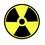# $f(n) = k^n$ for some $n$?

To be specific, there have been questions on this topic:

n = 0 to 5 (inclusive)

n = 0 to 6 (inclusive)

This wiki contains spoliers about these questions, so you are recommended to do them first before reading the rest of this note.

In these questions: Actually, please do those questions first.

In these questions, $k=2$ and $n = 0$ to $n=5$ and $n=0$ to $n=6$.

There's a lot of similarities, and even the answer is amazing, but I shall not spoil it.

Both questions are in the form "$f(n)$ is a $p$-degree polynomial. When $q = 0,1,2,\ldots,p$, $f(q) = k^q$. What is $f(x)$?"

These questions are a special case of this form, with $k = 2$ and x = 2*q+1. The answer is also very similar, f(x) = 2^(2*q).

I would like to know if this can be generalised for q and also, k, and if so, what values of x can create these values.

I'll work on it when I have time. Which doesn't come a lot.Note by Aloysius Ng
5 years, 5 months ago

This discussion board is a place to discuss our Daily Challenges and the math and science related to those challenges. Explanations are more than just a solution — they should explain the steps and thinking strategies that you used to obtain the solution. Comments should further the discussion of math and science.

When posting on Brilliant:

• Use the emojis to react to an explanation, whether you're congratulating a job well done , or just really confused .
• Ask specific questions about the challenge or the steps in somebody's explanation. Well-posed questions can add a lot to the discussion, but posting "I don't understand!" doesn't help anyone.
• Try to contribute something new to the discussion, whether it is an extension, generalization or other idea related to the challenge.

MarkdownAppears as
*italics* or _italics_ italics
**bold** or __bold__ bold
- bulleted- list
• bulleted
• list
1. numbered2. list
1. numbered
2. list
Note: you must add a full line of space before and after lists for them to show up correctly
paragraph 1paragraph 2

paragraph 1

paragraph 2

[example link](https://brilliant.org)example link
> This is a quote
This is a quote
    # I indented these lines
# 4 spaces, and now they show
# up as a code block.

print "hello world"
# I indented these lines
# 4 spaces, and now they show
# up as a code block.

print "hello world"
MathAppears as
Remember to wrap math in $$ ... $$ or $ ... $ to ensure proper formatting.
2 \times 3 $2 \times 3$
2^{34} $2^{34}$
a_{i-1} $a_{i-1}$
\frac{2}{3} $\frac{2}{3}$
\sqrt{2} $\sqrt{2}$
\sum_{i=1}^3 $\sum_{i=1}^3$
\sin \theta $\sin \theta$
\boxed{123} $\boxed{123}$

Sort by:

It can be done.

${ 3 }^{ n }={ 2 }^{ 0 }\left( \begin{matrix} n \\ 0 \end{matrix} \right) +{ 2 }^{ 1 }\left( \begin{matrix} n \\ 1 \end{matrix} \right) +{ 2 }^{ 2 }\left( \begin{matrix} n \\ 2 \end{matrix} \right) +\cdots +{ 2 }^{ n-1 }\left( \begin{matrix} n \\ n-1 \end{matrix} \right) +2^{ n }\left( \begin{matrix} n \\ n \end{matrix} \right)$

- 5 years, 4 months ago